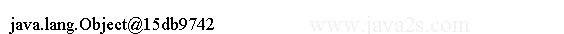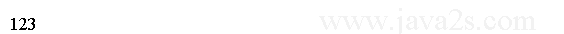Java Object Oriented Design - Java Object toString

The string representation of an object should contain enough information about the state of the object in a readable format.

The toString() method of the Object class represents the object of a class in a string.

The Object class provides a default implementation of the toString() method. It returns a string in the following format:

```<fully qualified class name>@<hash code of object in hexadecimal>
```

Example

Consider the following code and its output. You may get a different output.

```public class Main{
public static void main(String[] argv){
Object obj  = new Object();
String objStr = obj.toString();
System.out.println(objStr);
}
}
```

The code above generates the following result.Example 2

The following code shows how to create your own toString method.

```public class Main{
public static void main(String[] argv){
MyClass obj  = new MyClass(123);
String objStr = obj.toString();// w  ww.  jav a  2s . co  m
System.out.println(objStr);
}
}
class MyClass {
private int value;

public MyClass(int value) {
this.value = value;
}

public void setValue(int value) {
this.value = value;
}

public int getValue() {
return value;
}

/* override toString() method of the Object class */
public String toString() {
// Return the stored value as a string
String str = String.valueOf(this.value);
return str;
}
}
```

The code above generates the following result.Note

You need to make sure it is declared public, its return type is String, and it does not take any parameters.

The toString() method of a class is very important. Java calls the toString() method automatically when it needs a string representation of the object.

Two such situations that are worth mentioning:

When you concatenate a string and an object like

```String str = "Hello" + new Point(10, 20);
```

Java calls the toString() method on the Point object and concatenate the returned value to the "Hello" string.

The above statement is the same as the following one:

```String str = "Hello" + new Point(10, 20).toString();
```